NCERT Solutions: Coordinate Geometry (Exercise 3.1 & 3.2)

# Coordinate Geometry (Exercise 3.1 & 3.2) NCERT Solutions - Mathematics (Maths) Class 9

### Exercise 3.1

Q1. How will you describe the position of a table lamp on your study table to another person?
Ans: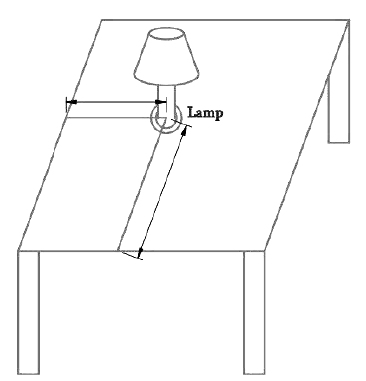The above figure is a 3-D shows a study table, on which a study lamp is placed.
Now let us consider only the top surface of the table which becomes a 2-D rectangle figure.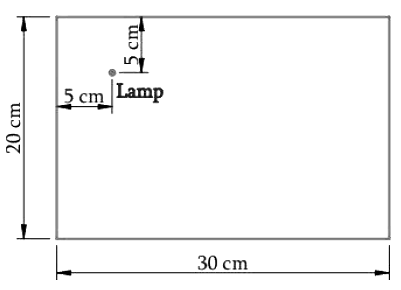From the Figure Above,

• Consider the lamp on the table as a point
• Consider the table as a 2-D plane.

The table has a shorter side (20 cm) and a longer side (30 cm).

• Let us measure the distance of the lamp from the shorter side and the longer side.
• Let us assume
• Distance of the lamp from the shorter side is 5 cm.
• Distance of the lamp from the longer side is 5 cm.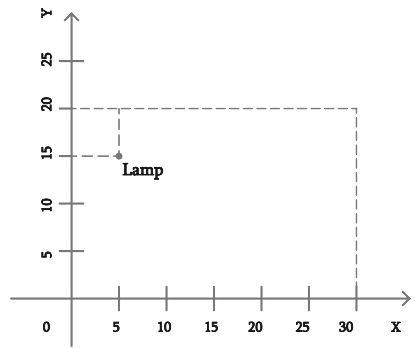Therefore, we can conclude that the position of the lamp on the table can be described with respect to the order of the axes as (5,15).

Q2. (Street Plan): A city has two main roads which cross each other at the center of the city.
These two roads are along the North–South direction and East– West direction.
All the other streets of the city run parallel to these roads and are 200 m apart. There are 5 streets in each direction. Using 1cm = 200 m, draw a model of the city on your notebook. Represent the roads/streets by single lines. There are many cross- streets in your model. A particular cross-street is made by two streets, one running in the North–South direction and another in the East– West direction. Each cross street is referred to in the following manner:
If the 2nd street running in the North–South direction and 5th in the East–West direction meet at some crossing, then we will call this cross-street (2, 5).
Using this convention, find:
(i) How many cross - streets can be referred to as (4, 3).
(ii) How many cross - streets can be referred to as (3, 4).
Ans: Let us draw two perpendicular lines as the two main roads of the city that cross each other at the center. Let us mark them as North–South and East–West.
As given in the question, let us take the scale as 1cm = 200 m.
Draw five streets that are parallel to both the main roads (which intersect), to get the given below figure.
Street plan is as shown in the figure:(i) There is only one cross street, which can be referred as (4, 3).
(ii) There is only one cross street, which can be referred as (3, 4).

### Exercise 3.2

Q1. Write the answer of each of the following questions:
(i) What is the name of horizontal and the vertical lines drawn to determine the position of any point in the Cartesian plane?
(ii) What is the name of each part of the plane formed by these two lines?
(iii) Write the name of the point where these two lines intersect.
Ans: (i) The horizontal line that is drawn to determine the position of any point in the Cartesian plane is called as x-axis. The vertical line that is drawn to determine the position of any point in the Cartesian plane is called as y-axis.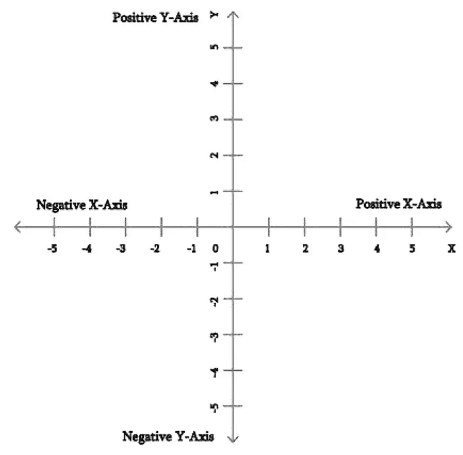(ii) The name of each part of the plane that is formed by x-axis and y-axis is called as quadrant.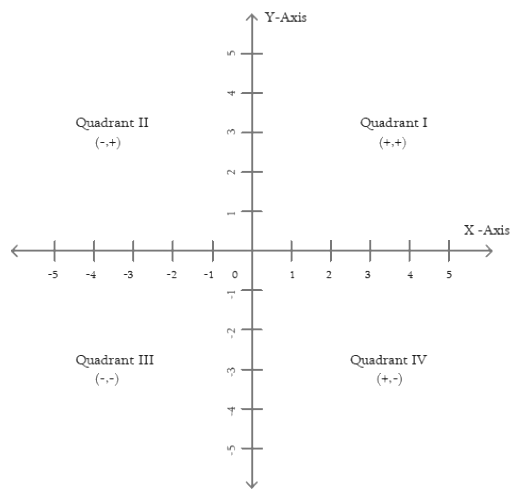(iii) The point, where the x-axis and the y-axis intersect is called as origin (O).

Q2. See the given figure, and write the following:
(i) The coordinates of B.
(ii) The coordinates of C.
(iii) The point identified by the coordinates (–3, –5).
(iv) The point identified by the coordinates (2, –4).
(v) The abscissa of the point D.
(vi) The ordinate of the point H.
(vii) The coordinates of the point L.
(viii) The coordinates of the point M.
Ans: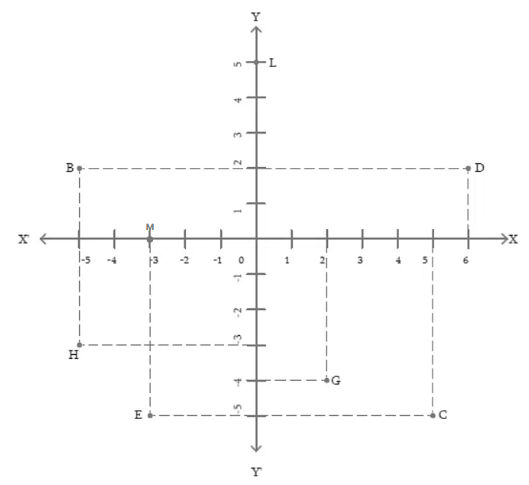From the Figure above,
(i) The co-ordinates of point B is the distance of point B from x-axis and y-axis.
Therefore, the co-ordinates of point B are (–5, 2).
(ii) The co-ordinates of point C is the distance of point C from x-axis and y-axis.
Therefore, the co-ordinates of point C are (5, –5).
(iii) The point that represents the co-ordinates (–3, –5) is E.
(iv) The point that represents the co-ordinates (2, –4) is G.
(v) The abscissa of point D is the distance of point D from the y-axis. Therefore, the abscissa of point D is 6.
(vi) The ordinate of point H is the distance of point H from the x-axis. Therefore, the abscissa of point H is –3.
(vii) The co-ordinates of point L in the above figure is the distance of point L from x-axis and y-axis. Therefore, the co-ordinates of point L are (0, 5).
(viii) The co-ordinates of point M in the above figure is the distance of point M from x-axis and y-axis. Therefore, the co-ordinates of point M are (–3, 0).

The document Coordinate Geometry (Exercise 3.1 & 3.2) NCERT Solutions | Mathematics (Maths) Class 9 is a part of the Class 9 Course Mathematics (Maths) Class 9.
All you need of Class 9 at this link: Class 9

## Mathematics (Maths) Class 9

42 videos|378 docs|65 tests

## FAQs on Coordinate Geometry (Exercise 3.1 & 3.2) NCERT Solutions - Mathematics (Maths) Class 9

 1. What is coordinate geometry?Ans. Coordinate geometry is a branch of mathematics that deals with the study of geometric figures using a coordinate system. It involves representing points, lines, curves, and shapes on a plane using numerical coordinates.
 2. How is the coordinate plane divided?Ans. The coordinate plane is divided into four quadrants, namely the first quadrant, second quadrant, third quadrant, and fourth quadrant. These quadrants help locate points on the plane by using positive and negative values of the x and y coordinates.
 3. What is the formula for the distance between two points in coordinate geometry?Ans. The formula for the distance between two points (x1, y1) and (x2, y2) in coordinate geometry is given by the distance formula: Distance = √[(x2 - x1)^2 + (y2 - y1)^2]
 4. How do you find the slope of a line in coordinate geometry?Ans. The slope of a line in coordinate geometry can be found using the formula: Slope = (change in y-coordinates) / (change in x-coordinates) = Δy / Δx
 5. What is the equation of a straight line in coordinate geometry?Ans. The equation of a straight line in coordinate geometry can be represented in the form y = mx + c, where m is the slope of the line and c is the y-intercept (the point where the line intersects the y-axis).

## Mathematics (Maths) Class 9

42 videos|378 docs|65 testsExplore Courses for Class 9 examSignup to see your scores go up within 7 days! Learn & Practice with 1000+ FREE Notes, Videos & Tests.
10M+ students study on EduRev
Track your progress, build streaks, highlight & save important lessons and more!
Related Searches

,

,

,

,

,

,

,

,

,

,

,

,

,

,

,

,

,

,

,

,

,

;